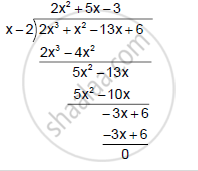Share

# Using the Reminder Theorem, Factorise of the Following Completely. 2x3 + X2 – 13x + 6 - Mathematics

Course

#### Question

Using the Reminder Theorem, factorise of the following completely.

2x3 + x2 – 13x + 6

#### Solution

Let f (x) = 2x3 + x2 − 13x + 6
For x = 2,
f(x) = f(2) = 2(2)3 + (2)2 − 13(2) + 6 = 16 + 4 − 26 + 6 = 0
Hence, (x − 2) is a factor of f(x).∴ 2x^3 + x^2 - 13x + 6 = (x-2) (2x^2 + 5x -3)

= (x-2)(2x^2 + 6x -x -3)

= (x-2) [2x(x + 3) -(x-3)]

= (x - 2)(x + 3) (2x - 1)

Is there an error in this question or solution?